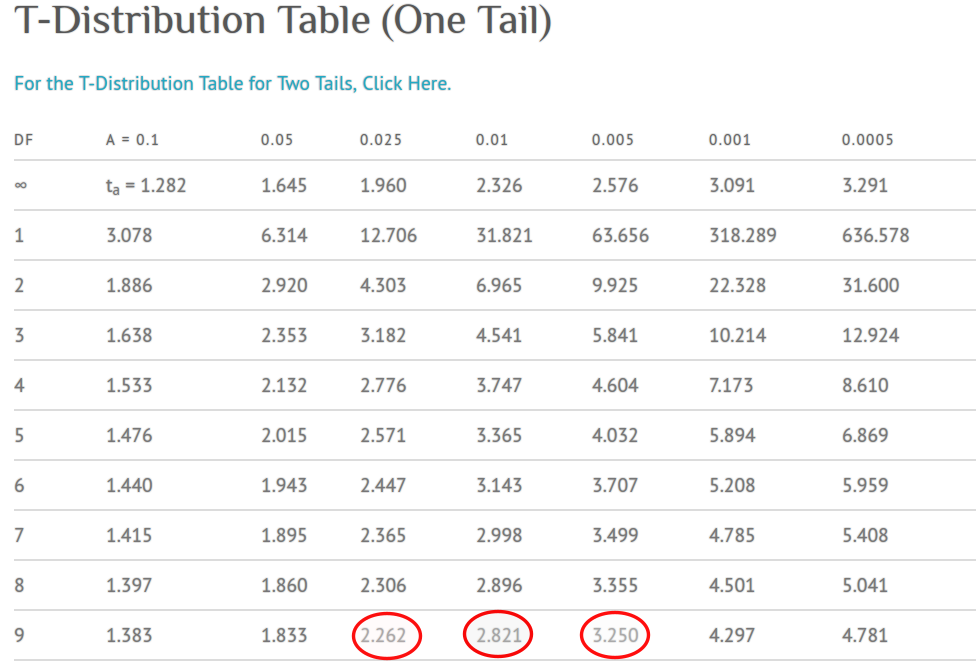# 第 24 章 比較 Comparisons

• 本章暫且只討論兩組之間的比較 (均值，方差，百分比)；
• 本章也只討論兩種類型的變量，連續型和二分類型變量
• 本章會介紹點估計 (point estimation)，信賴區間計算 (confidence intervals)，假設檢驗 (hypothesis testing)。

## 24.1 比較兩個均值 comparing two population means

### 24.1.1 當方差已知，且數據服從正態分佈 Z-test

$$Y_{1i} (i=1,2,\cdots, n_1); Y_{2i} (i=1,2,\cdots, n_2)$$ 表示兩個獨立且隨機的變量，他們來自兩個人羣 (1 和 2)，且各自的人羣均值爲 $$\mu_k$$，方差爲 $$\sigma_k^2$$

$E(Y_{ki})=\mu_k \text{ and Var}(Y_{ki}) = \sigma_k^2 \text{ for } k=1,2 \text{ and } i= 1,2,\cdots,n_k$

$\bar{Y}_k \sim N(\mu_k, \frac{\sigma_k^2}{n_k}) \text{ for } k=1,2$

$\begin{equation} \bar{Y}_2-\bar{Y}_1 \sim N(\mu_2-\mu_1, \frac{\sigma^2_2}{n_2}+\frac{\sigma^2_1}{n_1}) \end{equation} \tag{24.1}$

$\begin{equation} Z=\frac{\bar{Y}_2-\bar{Y}_1}{\sqrt{(\sigma_2^2/n_2)+(\sigma_1^2)/n_1}} \sim N(\frac{\mu_2-\mu_1}{\sqrt{(\sigma_2^2/n_2)+(\sigma_1^2)/n_1}},1) \end{equation} \tag{24.2}$

\begin{aligned} L & = (\bar{Y}_2 - \bar{Y}_1) + z_{\alpha/2}\sqrt{\frac{\sigma_2^2}{n_2}+\frac{\sigma_1^2}{n_1}}\\ U & = (\bar{Y}_2 - \bar{Y}_1) + z_{1-\alpha/2}\sqrt{\frac{\sigma_2^2}{n_2}+\frac{\sigma_1^2}{n_1}} \end{aligned}

$\begin{equation} (\bar{Y}_2 - \bar{Y}_1) \pm z_{1-\alpha/2}\sqrt{\frac{\sigma_2^2}{n_2}+\frac{\sigma_1^2}{n_1}} \end{equation} \tag{24.3}$

$\begin{equation} 2[1-\Phi(\frac{|\bar{y}_2-\bar{y}_1|}{\sqrt{(\sigma_2^2/n_2)+(\sigma_1^2/n_1)}})] \end{equation} \tag{24.4}$

1. 兩組的觀察數據 $$Y_{ki}$$ 均服從正態分佈；
2. 所有的觀察對象互相獨立；
3. 兩組數據來自的人羣的方差已知。

### 24.1.2 當方差未知，但是方差可以被認爲相等，且數據服從正態分佈 two sample $$t$$ test

$\bar{Y}_2-\bar{Y}_1 \sim N(\mu_2-\mu_1, \sigma^2(\frac{1}{n_2}+\frac{1}{n_1}))$

$\begin{equation} \hat\sigma^2 = \frac{(n_1-1)\hat\sigma^2_1+(n_2-1)\hat\sigma^2_2}{n_1+n_2-2} \end{equation} \tag{24.5}$

$\frac{(n_1+n_2-2)\hat\sigma^2_1}{\sigma^2} \sim \chi^2_{n_1+n_2-2}$

$T=\frac{(\bar{Y}_2-\bar{Y}_1) - (\mu_2-\mu_1)}{\hat\sigma\sqrt{(1/n_2)+(1/n_1)}} \sim t_{n_1+n_2-2}$

$(\bar{Y}_2-\bar{Y}_1) \pm t_{n_1+n_2-2, 1-\alpha/2}\hat\sigma\sqrt{(1/n_2)+(1/n_1)}$

### 24.1.3 練習

Age in months for walking alone
Active exercise group (i=1) Eight week control group (i=2)
9.00, 9.50, 9.75, 10.00, 13.00, 9.50 13.25, 11.50, 12.00, 13.50, 11.50
$$n_i$$ 6 5
$$\bar{Y}_i$$ 10.125 12.350
$$\hat\sigma_i$$ 1.447 0.962

$\hat\sigma^2 = \frac{(6-1)\times(1.447)^2+(5-1)\times(0.962)^2}{6+5-2} = \frac{14.172}{9} = 1.575$

$T=\frac{12.350-10.125}{\sqrt{1.575\times(1/5+1/6)}} = \frac{2.225}{0.76} = 2.928$24.1 中顯示統計量 $$t=2.928$$ 的單側 $$p$$ 值介於 $$0.01\sim0.005$$ 之間，所以此例的雙側 $$0.01 < p < 0.02$$

\begin{aligned} (\bar{Y}_2-\bar{Y}_1) &\pm t_{9, 0.975}\hat\sigma\sqrt{(1/n_2)+(1/n_1)} \\ = 2.225 &\pm 2.262 \times 0.76 = (0.51, 3.94) \end{aligned}

t.test(Walk$Age ~ Walk$Group, var.equal = TRUE)
##
##  Two Sample t-test
##
## data:  Walk$Age by Walk$Group
## t = -2.9, df = 9, p-value = 0.02
## alternative hypothesis: true difference in means is not equal to 0
## 95 percent confidence interval:
##  -3.9437 -0.5063
## sample estimates:
## mean in group exercise  mean in group control
##                  10.12                  12.35
summary(lm(Age ~ Group, data = Walk))
##
## Call:
## lm(formula = Age ~ Group, data = Walk)
##
## Residuals:
##    Min     1Q Median     3Q    Max
## -1.125 -0.738 -0.375  0.388  2.875
##
## Coefficients:
##              Estimate Std. Error t value Pr(>|t|)
## (Intercept)    10.125      0.512   19.77    1e-08 ***
## Groupcontrol    2.225      0.760    2.93    0.017 *
## ---
## Signif. codes:  0 '***' 0.001 '**' 0.01 '*' 0.05 '.' 0.1 ' ' 1
##
## Residual standard error: 1.25 on 9 degrees of freedom
## Multiple R-squared:  0.488,  Adjusted R-squared:  0.431
## F-statistic: 8.58 on 1 and 9 DF,  p-value: 0.0168

### 24.1.4 當方差未知，但是方差不可以被認爲相等，且數據服從正態分佈

$n^*=\frac{(\frac{\hat\sigma_1^2}{n_1}+\frac{\hat\sigma_2^2}{n_2})^2}{[\frac{(\hat\sigma_1^2/n_1)^2}{n_1-1}] + [\frac{(\hat\sigma_2^2/n_2)^2}{n_2-1}]}$

t.test(Walk$Age ~ Walk$Group, var.equal = FALSE)
##
##  Welch Two Sample t-test
##
## data:  Walk$Age by Walk$Group
## t = -3, df = 8.7, p-value = 0.01
## alternative hypothesis: true difference in means is not equal to 0
## 95 percent confidence interval:
##  -3.8879 -0.5621
## sample estimates:
## mean in group exercise  mean in group control
##                  10.12                  12.35

## 24.2 兩個人羣的方差比較

### 24.2.1 方差比值檢驗 variance ratio test

\begin{aligned} & \text{If } A\sim \chi_a^2 \text{ and } B \sim \chi_b^2 \text{ independently} \\ & \text{then } F = \frac{A/a}{B/b} \sim F_{a,b} \end{aligned}

var.test(Walk$Age~Walk$Group)
##
##  F test to compare two variances
##
## data:  Walk$Age by Walk$Group
## F = 2.3, num df = 5, denom df = 4, p-value = 0.4
## alternative hypothesis: true ratio of variances is not equal to 1
## 95 percent confidence interval:
##   0.2417 16.7226
## sample estimates:
## ratio of variances
##              2.264
leveneTest(Age ~ Group, data = Walk, center = median)
## Levene's Test for Homogeneity of Variance (center = median)
##       Df F value Pr(>F)
## group  1       0   0.95
##        9

### 24.2.2 信賴區間

$\begin{equation} (\frac{F}{F_{n_1-1,n_2-1, 0.975}} , \frac{F}{F_{n_1-1,n_2-1,0.025}}) \end{equation} \tag{24.6}$

$(\frac{F}{F_{n_1-1,n_2-1, 0.975}} , F\times F_{n_2-1,n_1-1,0.975})$

## 24.3 比較兩個百分比

### 24.3.1 兩個百分比差是否爲零的推斷 Risk difference

$$R_1, R_2$$ 爲兩種不同實驗的成功次數，每種實驗進行的次數分別是 $$n_1, n_2$$。類似地，令 $$P_1, P_2$$ 表示兩種實驗的觀察勝率。所以 $$R_1, R_2$$ 服從二項分佈：$$R_k \sim \text{Bin}(n_k, \pi_k) \text{ for } k=1,2$$。所以有：

\begin{aligned} & E(P_k) = \pi_k \text{ and Var}(P_k) = \frac{\pi_k(1-\pi_k)}{n_k} \\ & \text{For } k = 1,2 \text{ and } P_1, P_2 \text{ independent } \end{aligned}

$$n_k$$ 足夠大，每個百分比都可以根據中心極限定理用下面的正態分佈來近似：

$P_k \sim N(\pi_k, \frac{\pi_k(1-\pi_k)}{n_k}) \text{ for } k= 1,2$

$\begin{equation} P_2-P_1 \sim N(\pi_2-\pi_1, \frac{\pi_1(1-\pi_1)}{n_1}+\frac{\pi_2(1-\pi_2)}{n_2}) \end{equation} \tag{24.7}$

$(P_2-P_1) \pm z_{1-\alpha/2}\sqrt{\frac{P_1(1-P_1)}{n_1}+\frac{P_2(1-P_2)}{n_2}}$

\begin{aligned} & Z=\frac{P_2-P_1}{\sqrt{P(1-P)(\frac{1}{n_2}+\frac{1}{n_1})}} \sim N(0,1) \\ & \text{Where } P=\frac{R_1+R_2}{n_1+n_2} \text{ is the marginal probability of success} \\ & p\text{-value} = 2\times(1-\Phi\{ \frac{|P_2-P_1|}{\sqrt{P(1-P)(\frac{1}{n_2}+\frac{1}{n_1})}} \}) \end{aligned}

### 24.3.2 兩個百分比商是否爲 1 的推斷 relative risk/risk ratio

$\text{log} \frac{P_2}{P_1} \pm 1.96\times\sqrt{\frac{1-P_1}{R_1}+\frac{1-P_2}{R_2}}$